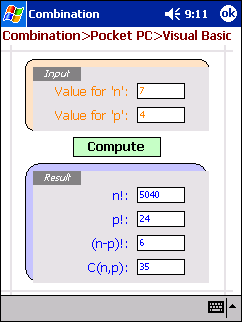Home > Pocket PC > Samples > Combination > Visual BasicCombination in Visual Basic

####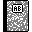Purpose

This is the eMbedded Visual Basic version of our combination application.

####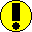Requirements

You need eMbedded Visual Tools and Pocket PC 2002 SDK (see tools section).

####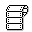Source code

Below is the .ebf file, which contains our main (and only) form along with its event handlers and the application's logic.

For .ebp (the project file), .vbw (the workspace) and .vb (the executable) please download the ZIP file.

```Rem Combination.ebf
Rem Pocket PC
Rem Visual Basic

Option Explicit

Private Function Factorial(n)
If n > 1 Then
Factorial = n * Factorial(n - 1)
Else: Factorial = 1
End If
End Function

Private Function Combination(n, p)
Combination = Factorial(n) / (Factorial(n - p) * Factorial(p))
End Function

Private Sub Compute()
Dim intN, intP As Integer

Rem Let's make sure the user input positive integers

On Error Resume Next
intN = n

On Error Resume Next
intP = p

If (intN <= 0) Or (intP <= 0) Then
MsgBox "Please do NOT input crap! Values HAVE to be positive integers..."
Exit Sub
End If

Rem Now, we do the job
factorialN.Text = Factorial(n)
factorialP.Text = Factorial(p)
factorialNP.Text = Factorial(n - p)
combinationNP.Text = Combination(n, p)
End Sub

Private Sub Compute_Click()
Rem User clicked on 'Compute' button, so we DO compute
Compute
End Sub

Private Sub Form_OKClick()
Rem User clicked OK to close the application - Time to say good bye
App.End
End Sub
```

####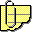Comments

See for yourself how compact this source code is. The embedded version of Visual Basic benefits from the model and features of the desktop version of VB. It is thus UI-oriented in its way of guiding you to develop an app.

The core functions are located in Factorial(), Combination() and Compute(). You'll notice that in most of our other samples, we used Go instead of Compute. We had to use Compute here because Go is a reserved keyword in VB.

Note that there's hardly any overhead for system or UI stuff.